# Relative Error Formula in Microsoft Excel

In this article, we will learn how many errors in Excel formula and what is formula in Excel to ignore the error.

We are having 7 types of Microsoft Excel Errors: - #VALUE!, #REF!, #DIV/0!, #NUM!, #NAME?, #NULL!,  and #N/A. Now, we will learn how to use excel formula to avoid the error.

Let’s take an example to understand all types of error.

#VALUE! Error

This error displays when a value used in a formula is of the wrong data type.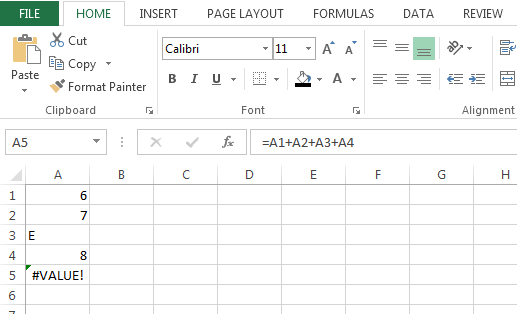Change the value of cell A3 or use a function to ignore cells that contain text.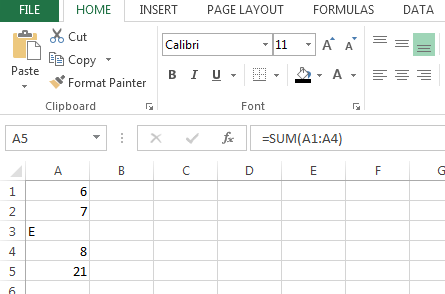#DIV/0! Error

This error comes when we divide the value to zero or empty cell.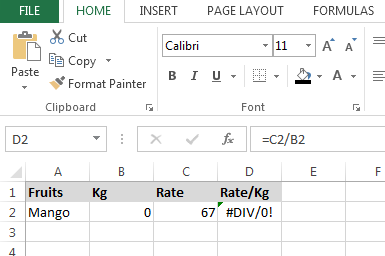To ignore the error use IFERROR formula, follow below given steps:-

• Enter the formula in cell E2.
• =IFERROR(C2/B2,"")
• Press Enter on your keyboard.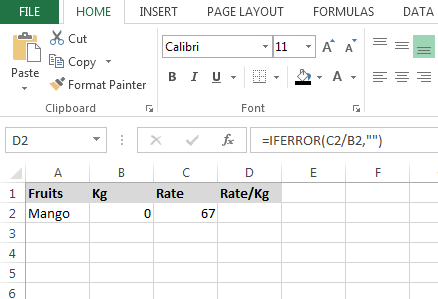#NUM! Error

This error occurs when we supply a non-valid number to a function argument. e.g. Using a negative number when a positive is required or using any symbol with the number.

#REF! Error

This error appears when we move or delete cell caused an invalid cell reference or function is returning reference error.

In the below shown example to return the rate/kg we have divided cell C2 to cell B2. When we will delete the cell B2 then this formula will give the #REF! Error.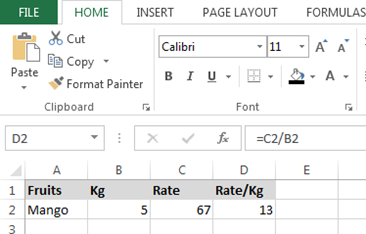#NAME? Error

This error occurs when we commit mistake in the spelling of a formula name.

Let’s understand to example:-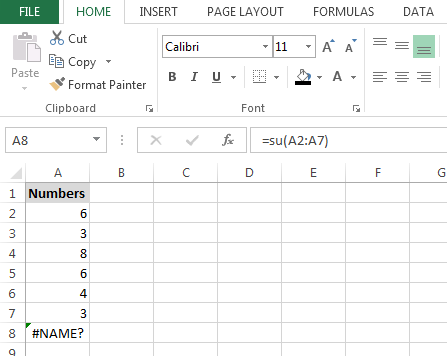#NULL! Error

This error occurs when the ranges in the formula do not intersect.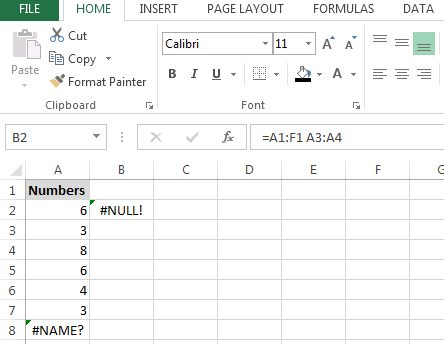#N/A Error

This error occurs when we match the two values and Excel cannot find a match. There are so many ways to correct this error.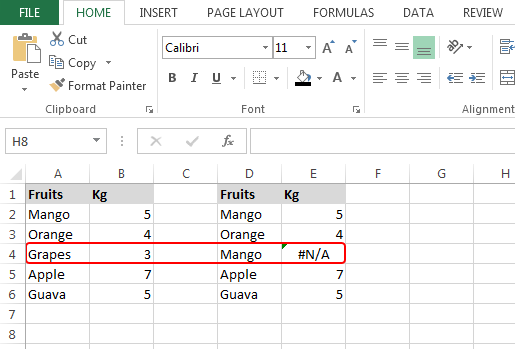Terms and Conditions of use

The applications/code on this site are distributed as is and without warranties or liability. In no event shall the owner of the copyrights, or the authors of the applications/code be liable for any loss of profit, any problems or any damage resulting from the use or evaluation of the applications/code.